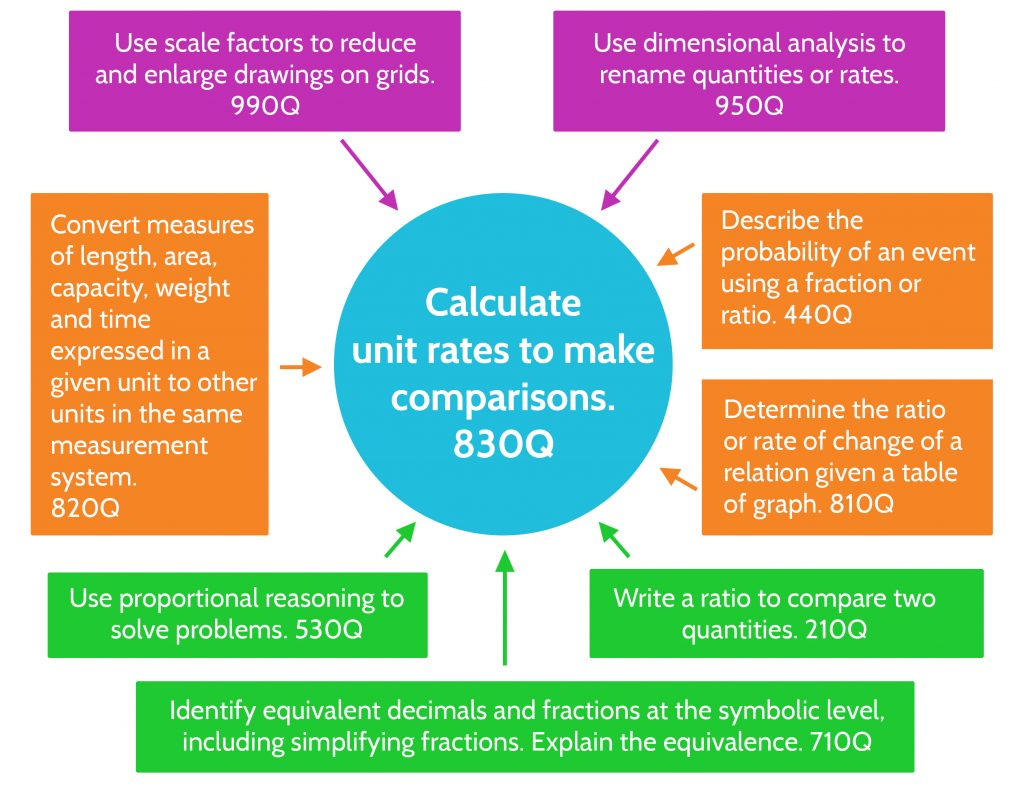Mathematical skills and concepts build upon one another. A child’s readiness to learn a specific skill or concept depends on having learned other skills or concepts first.

The Quantile® Framework for Mathematics has defined almost 500 mathematics skills and/or concepts. Each of these concepts has a measure, and each measure shows how difficult one skill is in relation to the others.

The description of a skill and its Quantile® measure is called a Quantile Skill and Concept (QSC). The table below shows a few of these skills and their measures. As difficulty, or demand, of the skill increases, so does the Quantile measure.

The QSCs connect to each other forming an enormous web of mathematics skills and concepts related through their content and their measures. This web of content spans content from kindergarten through secondary school mathematics.

## Examples of QSCsDescription of Skill or Concept Quantile Measure Solve quadratic inequalities graphically or algebraically. 1250Q Use properties of circles to solve problems involving arcs formed by central angles or inscribed angles. 1140Q Solve linear inequalities using the properties of inequality. 980Q Divide two fractions or a fraction and a whole number. 870Q Solve problems involving elapsed time. 450Q Identify and name: hexagon, trapezoid, parallelogram, and rhombus. 250Q

## Quantile Knowledge Clusters

Learning math isn’t a linear process. Some concepts can’t be learned until two or three others are mastered. And, when certain concepts are mastered, there are many directions you can go next.

That’s how we’ve structured QSCs. Each QSC concepts relates to other QSCs that are prerequisite concepts that students need to understand to progress in their study of mathematics.

These relationships form a Knowledge Cluster. Knowledge Clusters show the connections between mathematical skills and give their relative difficulty to one another using the Quantile scale. For example, below is the Knowledge Cluster for the math skill unit rate. This QSC has a Quantile measure of 830Q, and there are many QSCs that relate to it.

• Prerequisites are shown in green. They generally have lower Quantile measures than the skill the student is trying to learn.
• Supplemental skills, in orange, are related to learning about unit rate but are not dependent on learning or having learned about it.
• Impending skills, in purple, are those which depend on understanding unit rate, and can be learned after the student has mastered unit rates.The QSCs connect to each other forming an enormous web of mathematics skills and concepts related through their content and their measures. This web of content spans content from kindergarten through secondary school mathematics.

## The Quantile Map

The Quantile map is a visual representation of how QSCs, Knowledge Clusters, and student Quantile measures work together.  It provides examples of how The Quantile Framework for Mathematics works at various difficulty levels on the Quantile scale from basic mathematics concepts at EM400Q to more advanced mathematics skills at 1600Q.